# Bernstein polynomials

(diff) ← Older revision | Latest revision (diff) | Newer revision → (diff)

Algebraic polynomials defined by the formula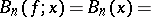Introduced by S.N. Bernshtein in 1912 (cf. ). The sequence of Bernstein polynomials converges uniformly to a functionon the segment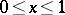ifis continuous on this segment. For a function which is bounded by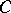,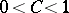, with a discontinuity of the first kind,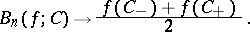The equationis valid ifis twice differentiable at the point. If the-th derivative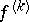of the function is continuous on the segment, the convergence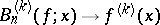is uniform on this segment. A study was made ([1b], ) of the convergence of Bernstein polynomials in the complex plane ifis analytic on the segment.

How to Cite This Entry:
Bernstein polynomials. Encyclopedia of Mathematics. URL: http://encyclopediaofmath.org/index.php?title=Bernstein_polynomials&oldid=13289
This article was adapted from an original article by P.P. Korovkin (originator), which appeared in Encyclopedia of Mathematics - ISBN 1402006098. See original article